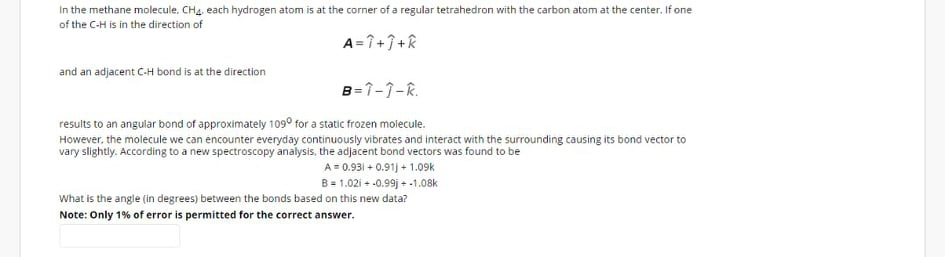# In the methane molecule. CH4, each hydrogen atom is at the corner of a regular tetrahedron with the carbon atom at the center. If one of the CH is in the direction of A=7+7+R and an adjacent C-H bond is at the direction B=1-1-R. results to an angular bond of approximately 109° for a static frozen molecule. However, the molecule we can encounter everyday continuously vibrates and interact with the surrounding causing its bond vector to vary slightly. According to a new spectroscopy analysis, the adjacent bond vectors was found to be A= 0.931 + 0.91) + 1.09k B= 1.02i + -0.99j + -1.08k What is the angle (in degrees) between the bonds based on this new data? Note: Only 1% of error is permitted for the correct answer.

Questionhelp_outlineImage TranscriptioncloseIn the methane molecule. CH4, each hydrogen atom is at the corner of a regular tetrahedron with the carbon atom at the center. If one of the CH is in the direction of A=7+7+R and an adjacent C-H bond is at the direction B=1-1-R. results to an angular bond of approximately 109° for a static frozen molecule. However, the molecule we can encounter everyday continuously vibrates and interact with the surrounding causing its bond vector to vary slightly. According to a new spectroscopy analysis, the adjacent bond vectors was found to be A= 0.931 + 0.91) + 1.09k B= 1.02i + -0.99j + -1.08k What is the angle (in degrees) between the bonds based on this new data? Note: Only 1% of error is permitted for the correct answer. fullscreen

### Want to see this answer and more?

Experts are waiting 24/7 to provide step-by-step solutions in as fast as 30 minutes!*

*Response times may vary by subject and question complexity. Median response time is 34 minutes for paid subscribers and may be longer for promotional offers.
Tagged in
Science
Physics

### Vectors and Scalars